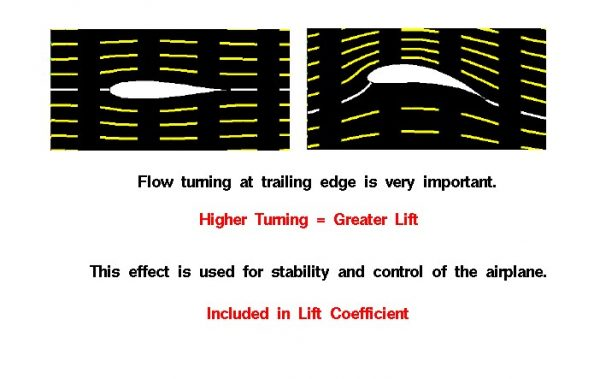# Effects of Shape on Lift Interactive## Shape

The amount of lift generated by an object depends on how much the flow is turned, which depends on the shape of the object. In general, the lift is a very complex function of the shape. Aerodynamicists model the shape effect by a lift coefficient which is normally determined through wind tunnel testing. For some simple shapes, we can develop mathematical equations to determine the lift coefficient. The simplest model, the two dimensional Kutta-Joukowski airfoil, is studied by undergraduate students. The FoilSim computer program provides the results of this analysis in a form readily usable by students. A result of the analysis shows that the greater the flow turning, the greater the lift generated by an airfoil.

## Airfoil

This slide shows the flow fields for two different airfoils. The airfoil on the left is a symmetric airfoil; the shapes above and below the white centerline are the same. The airfoil on the right is curved near the trailing edge. The yellow lines on each figure show the streamlines of flow from left to right. The left figure shows no net turning of the flow and produces no lift; the right figure shows a large amount of turning and generates a large amount of lift. The front portions of both airfoils are nearly identical. The aft portion of the right airfoil creates the higher turning.

## Wings

The example shown above explains why the aft portion of wings have hinged sections to control and maneuver an aircraft. Deflecting the aft section down produces a geometry similar to the figure on the right producing more lift. Similarly, if the aft section is deflected up, it creates less lift (or even negative lift). The ability to vary the amount of lift over a portion of the wing gives the pilot the ability to maneuver an aircraft.

• Elevator controls pitching motion.
• Rudder controls yawing motion.
• Ailerons control rolling motion.
Provide feedback